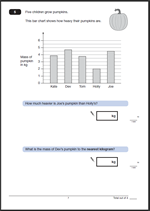# KS2 Maths 2011 Paper B: Question 5Here we have question 5 from the KS2 maths 2011 Paper B. There are two parts to the question, both based on interpreting a block graph.

The answer to 5a is:   2.5 or 2 1/2

One mark awarded for a correct answer.

Suggested method:

This is a straightforward graph question which should prove to be an easy mark to gain.

The important part of this question is to look carefully at the scale showing the mass of the pumpkin in kilos. Every other line is marked in kilos, so every other line must be a half kilo.

Joe’s pumpkin has a mass of 4.5 kg. Holly’s has a mass of 2 kg. The difference is 4.5 kg – 2 kg = 2.5 kg.

Note the use of mass rather than weight in this question. Children should be very familiar with this.

The answer to 5b is: 5 kg

One mark awarded for a correct answer.

Suggested method:

This is a rounding question. The first thing to do is to look at the mass of Dev’s pumpkin. It is between 4.5 kg and 5 kg. As it is more than 4.5 kilos the mass should be rounded up to 5 kg (to the nearest whole kilogram).

Question 5 from Paper B 2011

Question 5 answers and suggested method 2011 Paper B

Why not visit ks2-maths-sats.co.uk for free SATs papers and a great SAT revision programme?